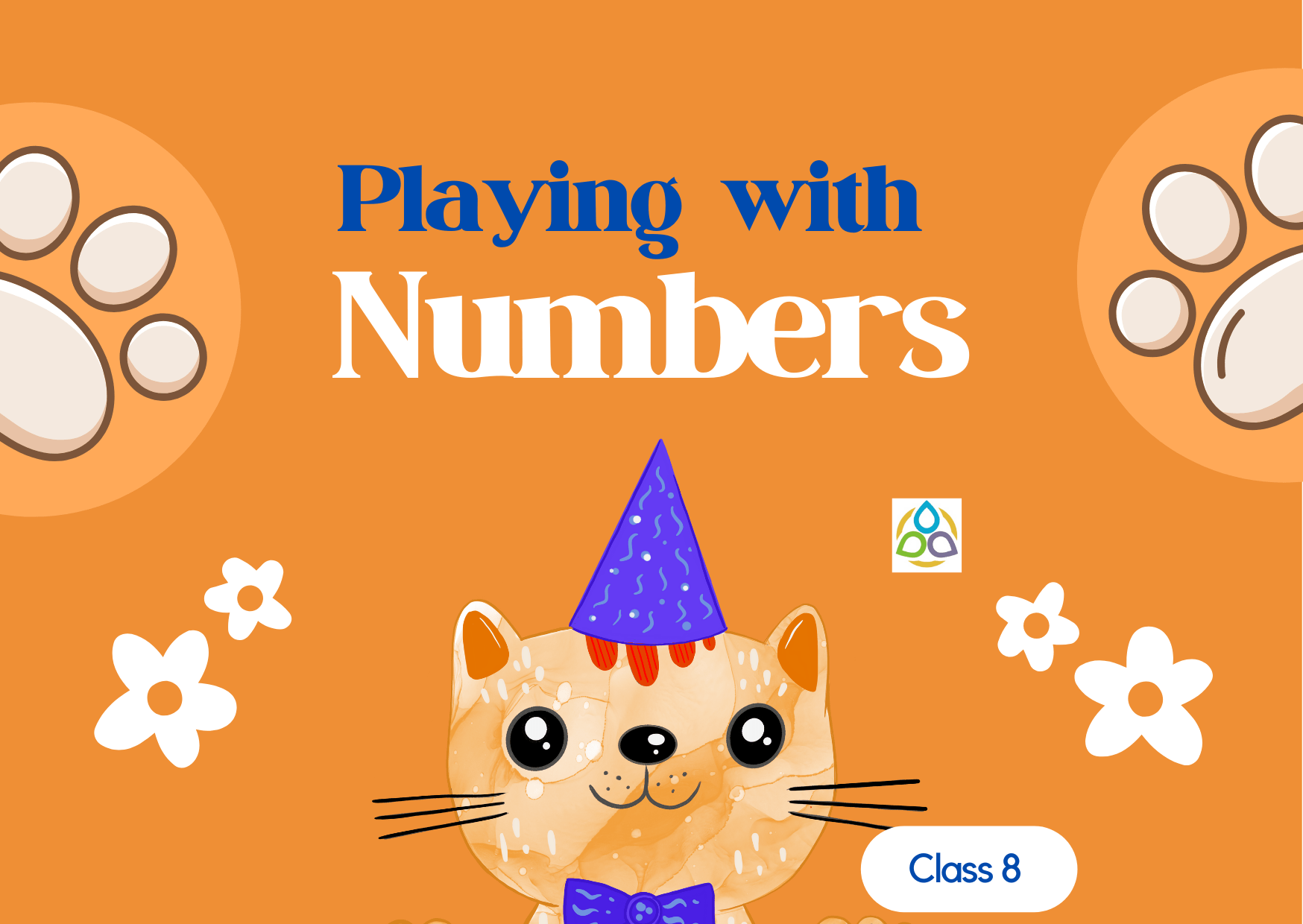# Playing with Numbers

Multiple Choice Questions

1. Generalised form of a two-digit number xy is

• (a) x + y
• (b) 10x + y
• (c) 10xy
• (d) 10y + x

2. Generalised form of a three-digit number xyz is

• (a) x + y + z
• (b) 100x + 10y + z
• (c) 100z + 10y + x
• (d) 100y + 10x + z

3. Generalised form of a four-digit number abdc is

• (a) 1000a + 100b + 10c + d
• (b) 1000a + 100c + 10b + d
• (c) 1000a + 100b + 10d + c
• (d) a × b × c × d

4. The usual form of 100a + b + 10c is

• (a) abc
• (b) cab
• (c) bac
• (d) acb

5. When written in usual form 100a + 10c + 9 is equal to

• (a) a9c
• (b) ac9
• (c) ca9
• (d) c9a

6. The usual form of 1000a + 10b + c is

• (a) abc
• (b) abc0
• (c) a0bc
• (d) ab0c

7. A six-digit number is formed by repeating a three-digit number. For example, 123123, 789789 etc. Any number of this form is divisible by

• (a) 7 only
• (b) 11 only
• (c) 13 only
• (d) 1001

8. The number abba, where a and b are digits and a > b is always divisible by

• (a) 15
• (b) 10
• (c) 9
• (d) 2

9. Let abc be a three-digit number. Then abccba is not divisible by

• (a) 9
• (b) 11
• (c) 18
• (d) 33

10. The sum of all the numbers formed by the digits x, y and z of the number xyz is divisible by

• (a) 11
• (b) 33
• (c) 37
• (d) 74

11. If the sum of digits of a number is divisible by three, then the number is always divisible by

• (a) 2
• (b) 3
• (c) 6
• (d) 9

1.DEB JYOTI MITRA says:

Mind Stretching Exercises

1.Math1089 says:

Thank you Sir

2.Sanjay Kumar Sharma says:

Amazing collection.

1.Math1089 says:

Thank you. Hope this will help the students.